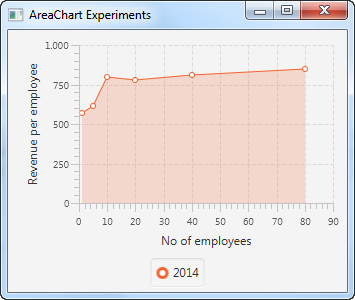# JavaFX AreaChart

 Jakob Jenkov Last update: 2016-05-29

The JavaFX AreaChart can draw area charts inside your JavaFX applications. An area chart is a line chart where the area below the lines are painted with color. The JavaFX AreaChart component is represented by the class `javafx.scene.chart.AreaChart` .

## AreaChart X Axis and Y Axis

A JavaFX AreaChart draws an area chart. An area chart is a two-dimensional graph, meaning the graph has an X axis and a Y axis. Area charts typically have two numerical axes. A numerical axis is represented by the JavaFX class `javafx.scene.chart.NumberAxis` .

You need to define the X axis and Y axis used by a `AreaChart`. Here is an example of creating two JavaFX `NumberAxis` instances:

```NumberAxis xAxis = new NumberAxis();
xAxis.setLabel("No of employees");

NumberAxis yAxis = new NumberAxis();
yAxis.setLabel("Revenue per employee");
```

## Creating an AreaChart

You create a JavaFX AreaChart component by creating an instance of the `AreaChart` class. You need to pass an X axis and Y axis to the `AreaChart` constructor. Here is a JavaFX `AreaChart` instantiation example:

```NumberAxis xAxis = new NumberAxis();
xAxis.setLabel("No of employees");

NumberAxis yAxis = new NumberAxis();
yAxis.setLabel("Revenue per employee");

AreaChart areaChart = new AreaChart(xAxis, yAxis);
```

## AreaChart Data Series

To get a JavaFX `AreaChart` component to display anything, you must provide it with a data series. A data series is a list of data points. Each data point contains an X value and a Y value. Here is an example of creating a data series and adding it to a `AreaChart` component:

```XYChart.Series dataSeries1 = new XYChart.Series();
dataSeries1.setName("2014");

```

First an `XYChart.Series` instance is created and given a name. Second, 6 `XYChart.Data` instances are added to the `XYChart.Series` object. Third, the `XYChart.Series` object is added to a `AreaChart` object.

It is possible to add multiple data series to the `AreaChart` . Just repeat the above code for additional data series.

## Adding an AreaChart to the Scene Graph

To make a JavaFX `AreaChart` visible you must add it to the JavaFX scene graph. This means adding the `AreaChart` to a `Scene` object or add the `AreaChart` to a layout component which is added to a `Scene` object.

Here is an example that adds an `AreaChart` to the JavaFX scene graph:

```package com.jenkov.javafx.charts;

import javafx.application.Application;
import javafx.scene.Scene;
import javafx.scene.chart.AreaChart;
import javafx.scene.chart.NumberAxis;
import javafx.scene.chart.XYChart;
import javafx.scene.layout.VBox;
import javafx.stage.Stage;

public class AreaChartExperiments extends Application {

@Override
public void start(Stage primaryStage) throws Exception {
primaryStage.setTitle("AreaChart Experiments");

NumberAxis xAxis = new NumberAxis();
xAxis.setLabel("No of employees");

NumberAxis yAxis = new NumberAxis();
yAxis.setLabel("Revenue per employee");

AreaChart areaChart = new AreaChart(xAxis, yAxis);

XYChart.Series dataSeries1 = new XYChart.Series();
dataSeries1.setName("2014");

VBox vbox = new VBox(areaChart);

Scene scene = new Scene(vbox, 400, 200);

primaryStage.setScene(scene);
primaryStage.setHeight(300);
primaryStage.setWidth(1200);

primaryStage.show();
}

public static void main(String[] args) {
Application.launch(args);
}
}

```

The application resulting from running this application would look similar to this:Tweet Jakob Jenkov
Featured VideosClose TOCAll TrailsTrail TOCPage TOCPreviousNext